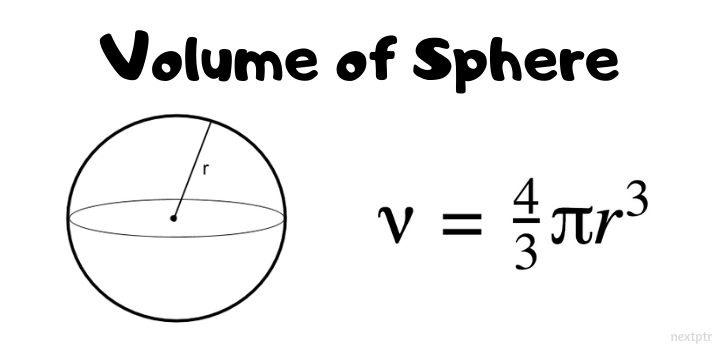# Returning an arrow function from a functionThe SphereVolumeFormula function returns an arrow function that computes and returns volume of a sphere of a specific radius `r`:

``````function SphereVolumeFormula(r) {
// returning an arrow function
return () => (4/3)*Math.PI*(r*r*r);
}

// Get a volume formula function for a sphere of radius 2
let formula = SphereVolumeFormula(2);
``````

What is the result of calling formula() ?

``````console.log(formula());
``````